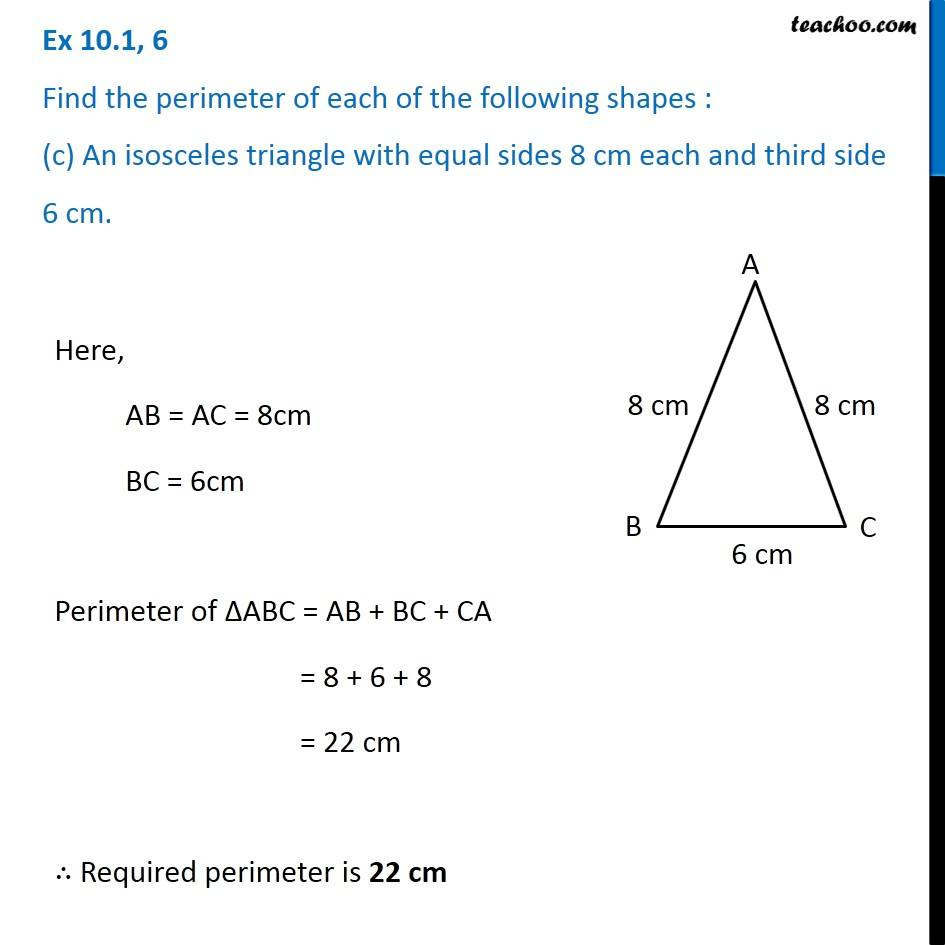Ex 10.1

Chapter 10 Class 6 Mensuration
Serial order wiseLearn in your speed, with individual attention - Teachoo Maths 1-on-1 Class

### Transcript

Ex 10.1, 6 Find the perimeter of each of the following shapes : (c) An isosceles triangle with equal sides 8 cm each and third side 6 cm. Here, AB = AC = 8cm BC = 6cm Perimeter of ∆ABC = AB + BC + CA = 8 + 6 + 8 = 22 cm ∴ Required perimeter is 22 cm